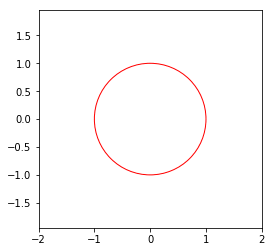#### matplotlib怎么画圆？

统计/机器学习 数据可视化 Python    浏览次数：4198        分享

0图不二   2019-03-19 11:46

##### 1个回答
5import matplotlib.pyplot as plt
plt.figure(figsize=(4, 4))
plt.axis('equal')
ax = plt.gca()
ax.set_xlim((-2, 2))
ax.set_ylim((-2, 2))
disk1 = plt.Circle((0, 0), 1, color='r', fill=False)
plt.show()
SofaSofa数据科学社区DS面试题库 DS面经okayguy   2019-03-20 09:54相关主题